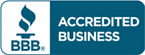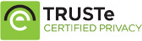# Test bank for Principles of Econometrics, 4th Edition R. Carter Hill

\$30.00

Principles of Econometrics, 4th Edition R. Carter Hill Test bankCategories: ,

## Description

Test bank for Principles of Econometrics, 4th Edition R. Carter Hill

Table of content
Chapter 1   Introducing Stata
Chapter 2   Simple linear regression
Chapter 3   Interval Estimation and Hypothesis Testing
Chapter 4   Prediction, Goodness-of-Fit and Modeling Issues
Chapter 5   Multiple Linear Regression
Chapter 6   Further Inference in the Multiple Regression Model
Chapter 7   Using Indicator Variables
Chapter 8   Heteroskedasticity
Chapter 9   Regression with Time-Series Data: Stationary Variables
Chapter 10  Random Regressors and Moment Based Estimation
Chapter 11   Simultaneous Equations Models
Chapter 12   Regression with Time-Series Data: Nonstationary Variables
Chapter 13   Vector Error Correction and Vector Autoregressive Models
Chapter 14   Time-Varying Volatility and ARCH Models
Chapter 15   Panel Data Models
Chapter 16   Qualitative and Limited Dependent Variable Models

## Reviews

There are no reviews yet.